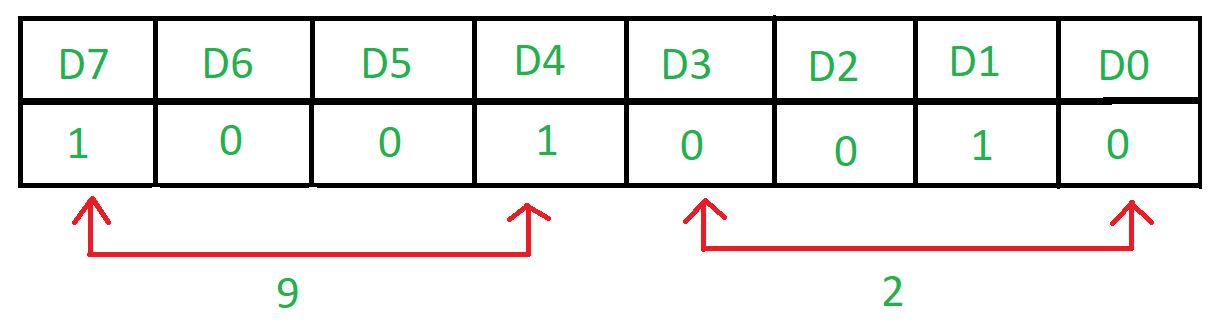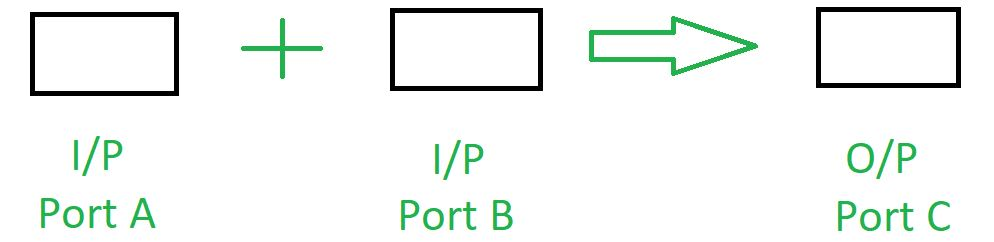# Interface 8255 with 8085 microprocessor for addition

Problem – Interface 8255 with 8085 microprocessor and write an assembly program that determines the addition of contents of port A and port B and stores the result in port C.

Example –Algorithm –

1. Construct the control word register
2. Input the data from port A and port B
3. Add the contents of port A and port B
4. Display the result in port C

Program –

Explanation –

• MVI A, 92H means that the value of the control register is 92.
```D7=1 as it is in I/O mode.
D6=0 & D5=0 as Port A is in m0 mode.
D4=1 as Port A is taking input.
D3=0 & D0=0 as Port C is not taking part.
D2=0 as mode of Port B is m0.
D1=1as Port B is taking the input.```
• OUT 83H putting the value of A in 83H which is the port number of the port control register.
• IN 80H taking input from 80H which is the port number of port A.
• MOV B, A copies the content of A register to B register.
• IN 81H takes input from 81H which is the port number of port B.
• ADD B add the contents of A register and B register.
• OUT 82H displays the result in 81H which is the port number of port C.
• RET return

• The 8255 is a versatile and widely used programmable peripheral interface that provides 24 input/output pins, allowing for a wide range of input and output operations.

• Using the 8255 allows for easy expansion of the microprocessor’s capabilities by providing additional input/output ports.

• The interface circuit provides an efficient method for performing addition operations by using the add instruction of the 8085 microprocessor and the input/output ports of the 8255.

• The interface circuit is relatively simple and can be easily implemented on a breadboard or printed circuit board.